# RD Sharma Solutions for Class 11 Maths Exercise 20.1 Chapter 20 - Geometric Progressions

This exercise mainly deals with problems based on geometric progression (G.P.) and the general term of a G.P. For students who face difficulty in understanding the concepts during class hours, the subject experts at BYJU’S have formulated the solutions in a simple language based on the student’s ability. Students can practice the examples and can solve exercise wise problems at ease, which boosts their confidence level before appearing for the board exam. RD Sharma Class 11 Maths Solutions is readily available in the pdf format for students to download easily and start practising offline.

## Download the pdf of RD Sharma Solutions for Class 11 Maths Exercise 20.1 Chapter 20 – Geometric Progression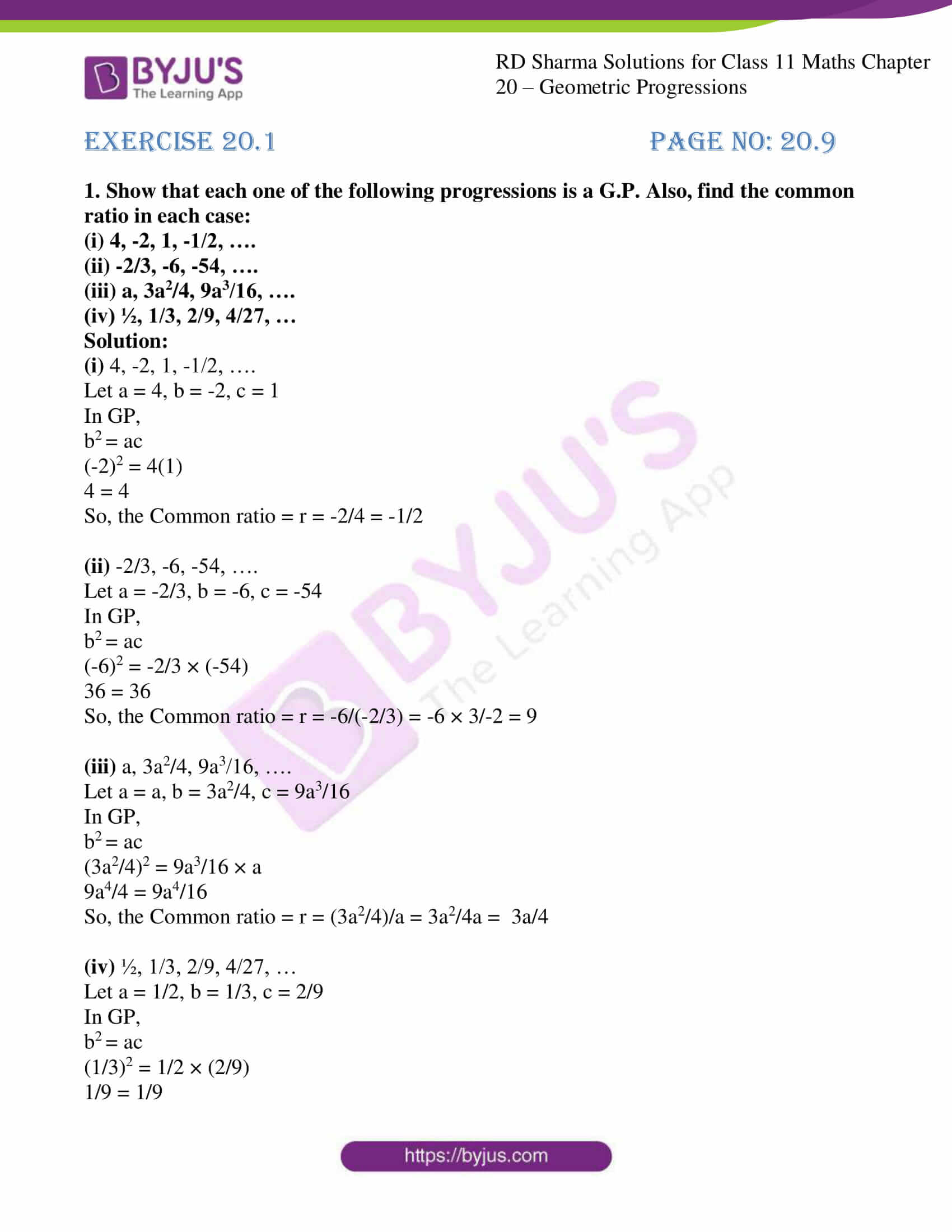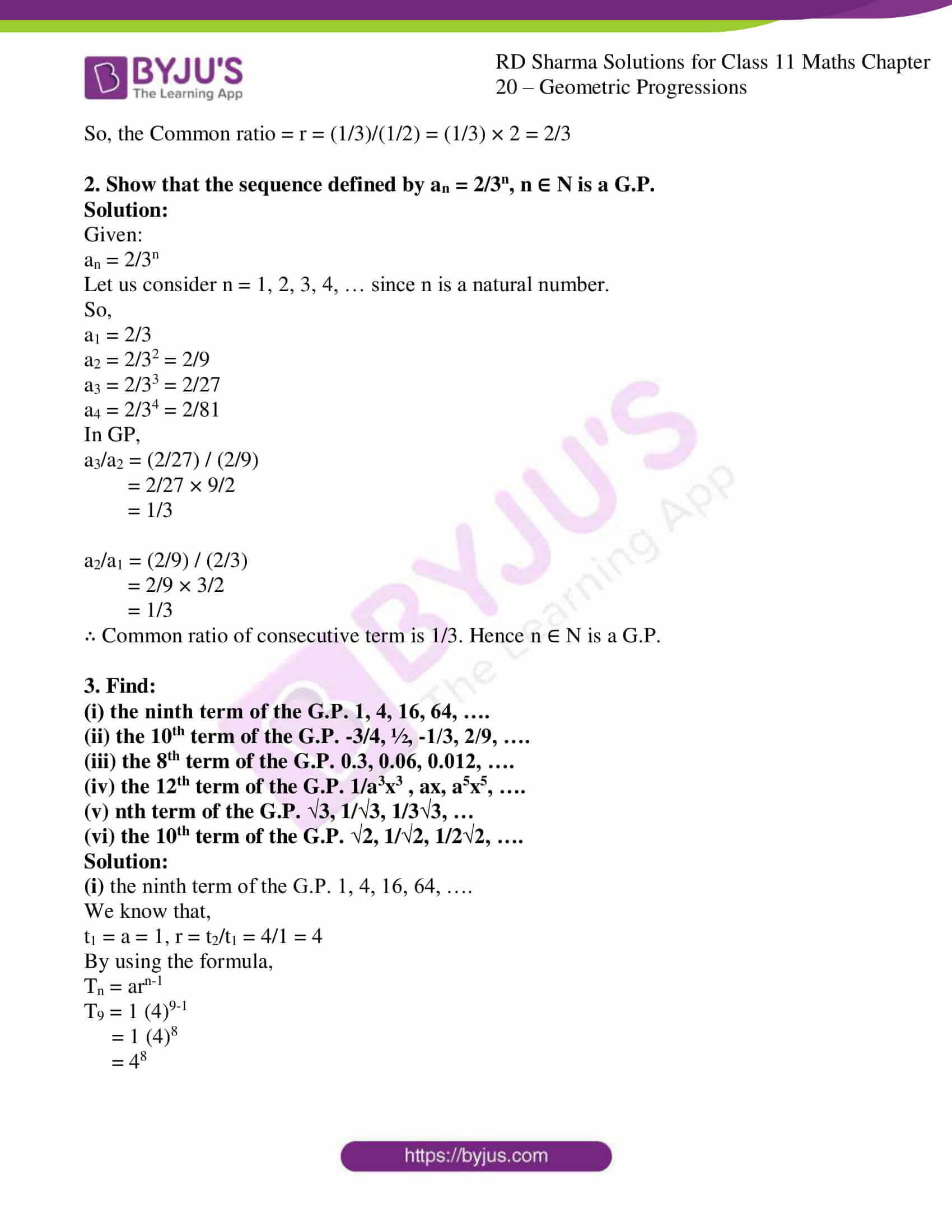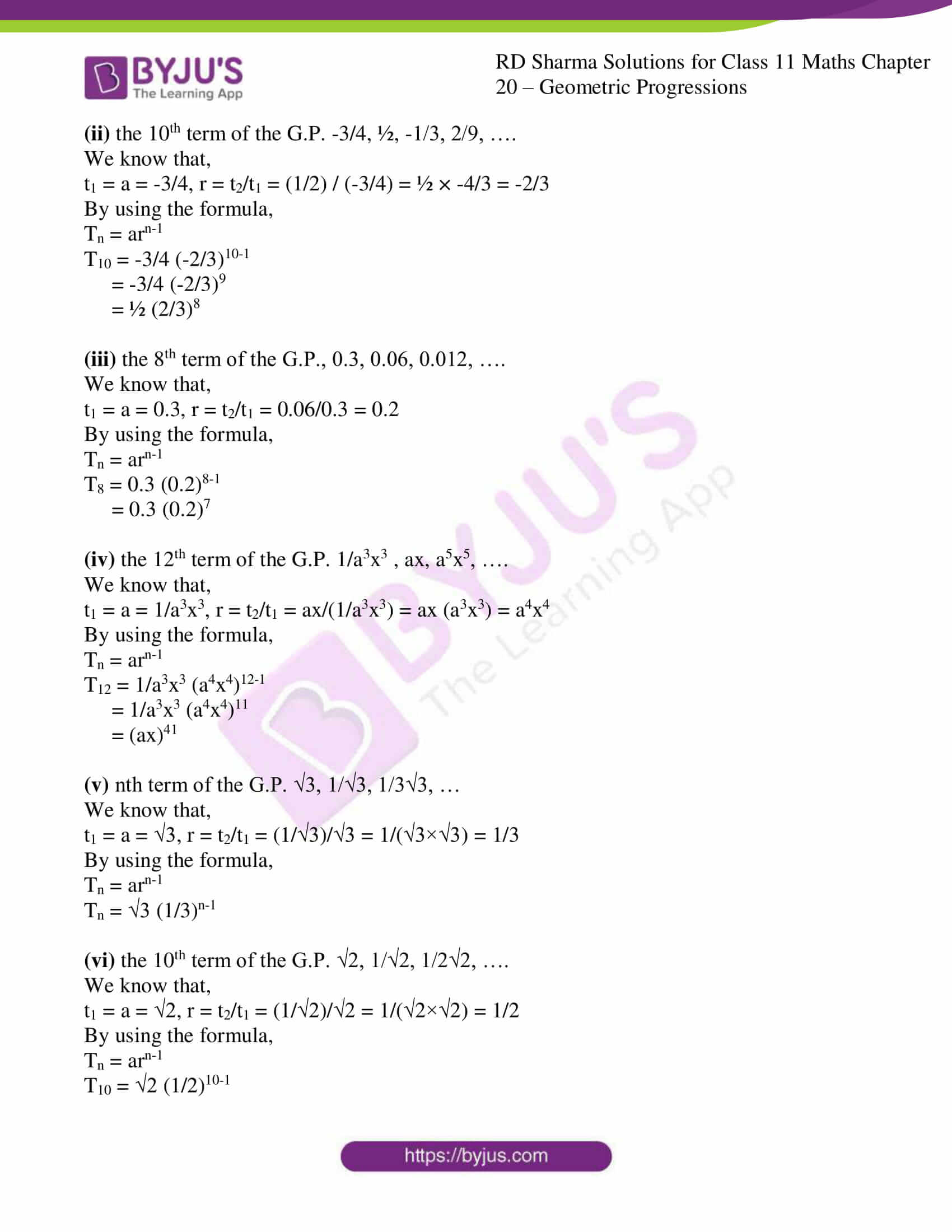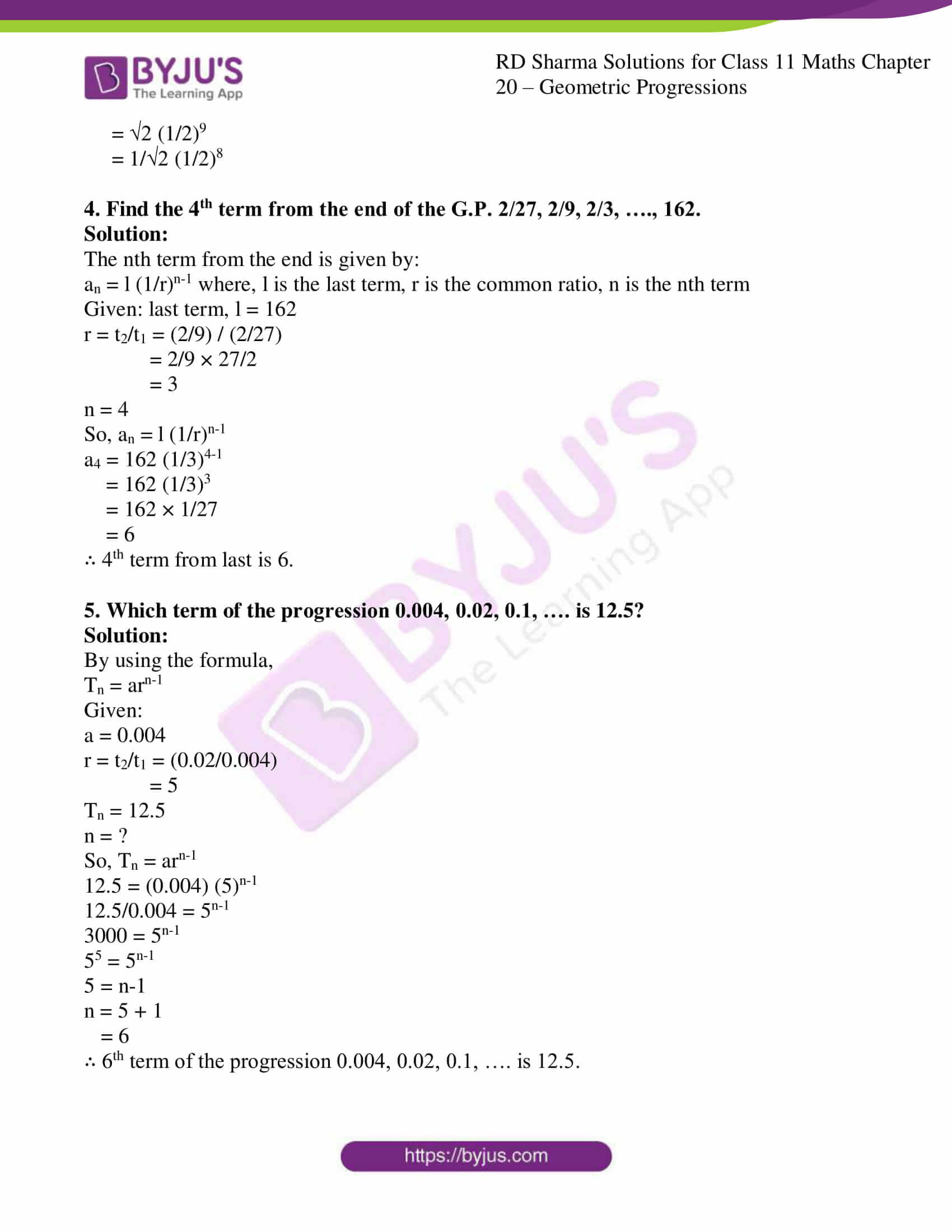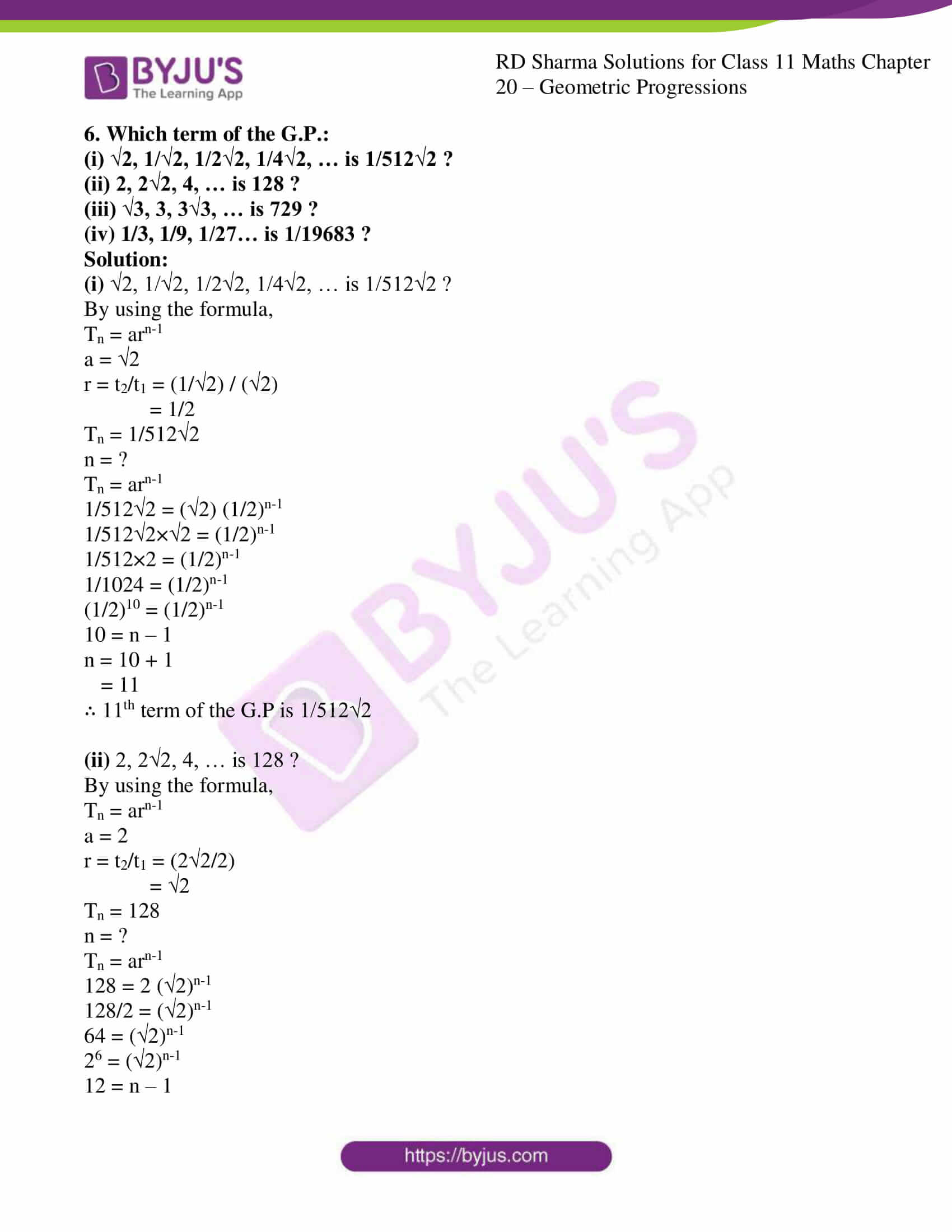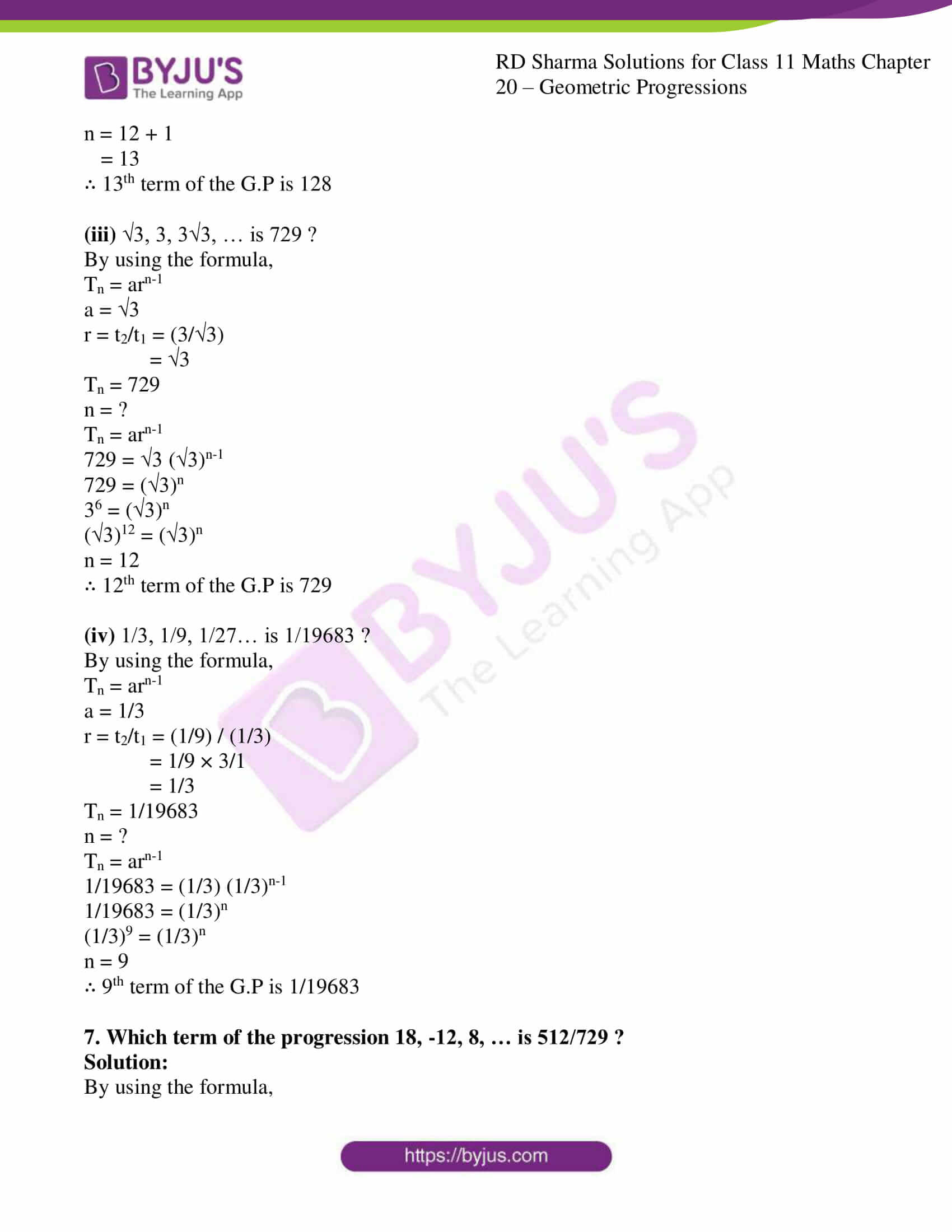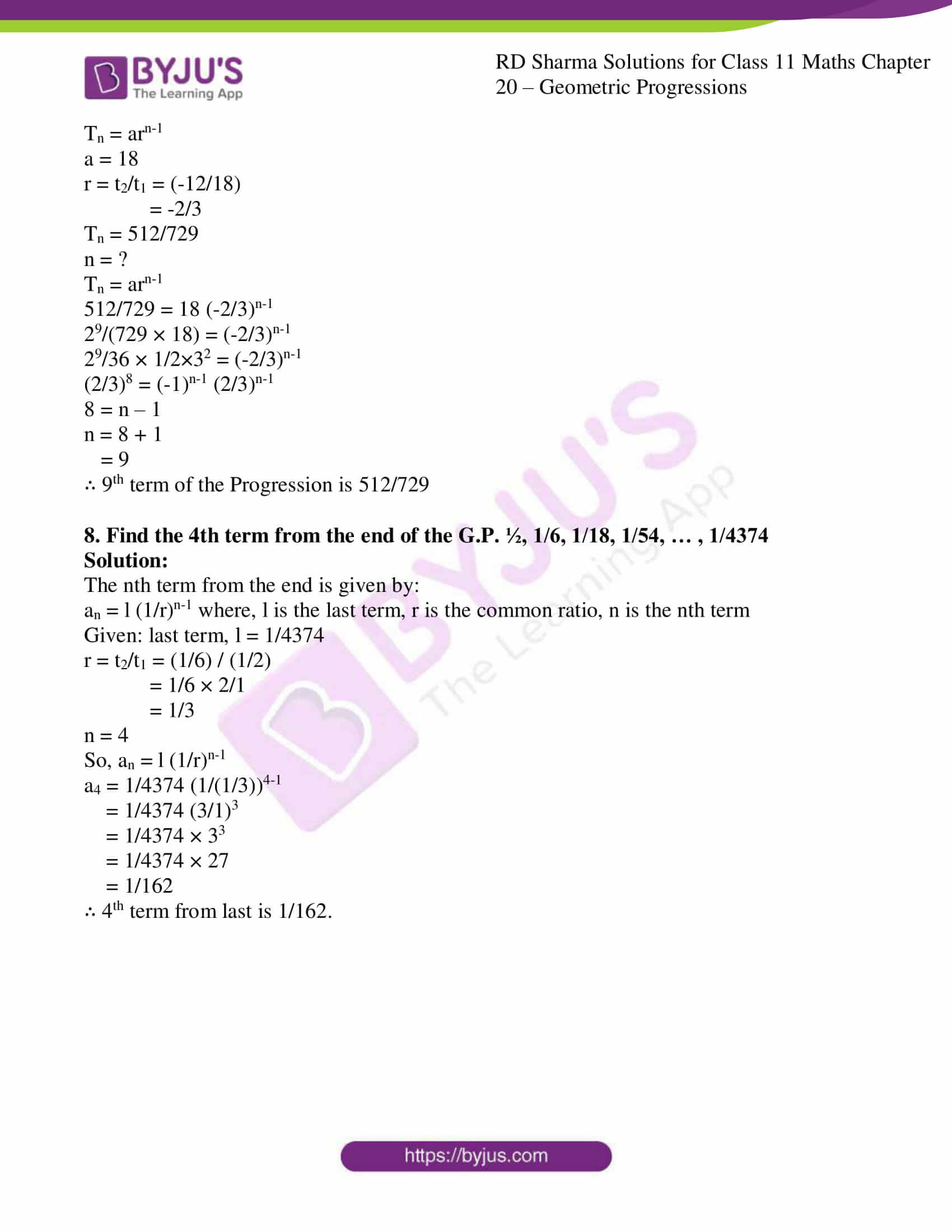### Also, access other exercises of RD Sharma Solutions for Class 11 Maths Chapter 20 – Geometric Progression

Exercise 20.2 Solutions

Exercise 20.3 Solutions

Exercise 20.4 Solutions

Exercise 20.5 Solutions

Exercise 20.6 Solutions

### Access answers to RD Sharma Solutions for Class 11 Maths Exercise 20.1 Chapter 20 – Geometric Progression

1. Show that each one of the following progressions is a G.P. Also, find the common ratio in each case:

(i) 4, -2, 1, -1/2, ….

(ii) -2/3, -6, -54, ….

(iii) a, 3a2/4, 9a3/16, ….

(iv) ½, 1/3, 2/9, 4/27, …

Solution:

(i) 4, -2, 1, -1/2, ….

Let a = 4, b = -2, c = 1

In GP,

b2 = ac

(-2)2 = 4(1)

4 = 4

So, the Common ratio = r = -2/4 = -1/2

(ii) -2/3, -6, -54, ….

Let a = -2/3, b = -6, c = -54

In GP,

b2 = ac

(-6)2 = -2/3 × (-54)

36 = 36

So, the Common ratio = r = -6/(-2/3) = -6 × 3/-2 = 9

(iii) a, 3a2/4, 9a3/16, ….

Let a = a, b = 3a2/4, c = 9a3/16

In GP,

b2 = ac

(3a2/4)2 = 9a3/16 × a

9a4/4 = 9a4/16

So, the Common ratio = r = (3a2/4)/a = 3a2/4a = 3a/4

(iv) ½, 1/3, 2/9, 4/27, …

Let a = 1/2, b = 1/3, c = 2/9

In GP,

b2 = ac

(1/3)2 = 1/2 × (2/9)

1/9 = 1/9

So, the Common ratio = r = (1/3)/(1/2) = (1/3) × 2 = 2/3

2. Show that the sequence defined by an = 2/3n, n ∈ N is a G.P.

Solution:

Given:

an = 2/3n

Let us consider n = 1, 2, 3, 4, … since n is a natural number.

So,

a1 = 2/3

a2 = 2/32 = 2/9

a3 = 2/33 = 2/27

a4 = 2/34 = 2/81

In GP,

a3/a2 = (2/27) / (2/9)

= 2/27 × 9/2

= 1/3

a2/a1 = (2/9) / (2/3)

= 2/9 × 3/2

= 1/3

∴ Common ratio of consecutive term is 1/3. Hence n ∈ N is a G.P.

3. Find:

(i) the ninth term of the G.P. 1, 4, 16, 64, ….

(ii) the 10th term of the G.P. -3/4, ½, -1/3, 2/9, ….

(iii) the 8th term of the G.P. 0.3, 0.06, 0.012, ….

(iv) the 12th term of the G.P. 1/a3x3 , ax, a5x5, ….

(v) nth term of the G.P. √3, 1/√3, 1/3√3, …

(vi) the 10th term of the G.P. √2, 1/√2, 1/2√2, ….

Solution:

(i) the ninth term of the G.P. 1, 4, 16, 64, ….

We know that,

t1 = a = 1, r = t2/t1 = 4/1 = 4

By using the formula,

Tn = arn-1

T9 = 1 (4)9-1

= 1 (4)8

= 48

(ii) the 10th term of the G.P. -3/4, ½, -1/3, 2/9, ….

We know that,

t1 = a = -3/4, r = t2/t1 = (1/2) / (-3/4) = ½ × -4/3 = -2/3

By using the formula,

Tn = arn-1

T10 = -3/4 (-2/3)10-1

= -3/4 (-2/3)9

= ½ (2/3)8

(iii) the 8th term of the G.P., 0.3, 0.06, 0.012, ….

We know that,

t1 = a = 0.3, r = t2/t1 = 0.06/0.3 = 0.2

By using the formula,

Tn = arn-1

T8 = 0.3 (0.2)8-1

= 0.3 (0.2)7

(iv) the 12th term of the G.P. 1/a3x3 , ax, a5x5, ….

We know that,

t1 = a = 1/a3x3, r = t2/t1 = ax/(1/a3x3) = ax (a3x3) = a4x4

By using the formula,

Tn = arn-1

T12 = 1/a3x3 (a4x4)12-1

= 1/a3x3 (a4x4)11

= (ax)41

(v) nth term of the G.P. √3, 1/√3, 1/3√3, …

We know that,

t1 = a = √3, r = t2/t1 = (1/√3)/√3 = 1/(√3×√3) = 1/3

By using the formula,

Tn = arn-1

Tn = √3 (1/3)n-1

(vi) the 10th term of the G.P. √2, 1/√2, 1/2√2, ….

We know that,

t1 = a = √2, r = t2/t1 = (1/√2)/√2 = 1/(√2×√2) = 1/2

By using the formula,

Tn = arn-1

T10 = √2 (1/2)10-1

= √2 (1/2)9

= 1/√2 (1/2)8

4. Find the 4th term from the end of the G.P. 2/27, 2/9, 2/3, …., 162.

Solution:

The nth term from the end is given by:

an = l (1/r)n-1 where, l is the last term, r is the common ratio, n is the nth term

Given: last term, l = 162

r = t2/t1 = (2/9) / (2/27)

= 2/9 × 27/2

= 3

n = 4

So, an = l (1/r)n-1

a4 = 162 (1/3)4-1

= 162 (1/3)3

= 162 × 1/27

= 6

∴ 4th term from last is 6.

5. Which term of the progression 0.004, 0.02, 0.1, …. is 12.5?

Solution:

By using the formula,

Tn = arn-1

Given:

a = 0.004

r = t2/t1 = (0.02/0.004)

= 5

Tn = 12.5

n = ?

So, Tn = arn-1

12.5 = (0.004) (5)n-1

12.5/0.004 = 5n-1

3000 = 5n-1

55 = 5n-1

5 = n-1

n = 5 + 1

= 6

∴ 6th term of the progression 0.004, 0.02, 0.1, …. is 12.5.

6. Which term of the G.P.:

(i) √2, 1/√2, 1/2√2, 1/4√2, … is 1/512√2 ?

(ii) 2, 2√2, 4, … is 128 ?

(iii) √3, 3, 3√3, … is 729 ?

(iv) 1/3, 1/9, 1/27… is 1/19683 ?

Solution:

(i) √2, 1/√2, 1/2√2, 1/4√2, … is 1/512√2 ?

By using the formula,

Tn = arn-1

a = √2

r = t2/t1 = (1/√2) / (√2)

= 1/2

Tn = 1/512√2

n = ?

Tn = arn-1

1/512√2 = (√2) (1/2)n-1

1/512√2×√2 = (1/2)n-1

1/512×2 = (1/2)n-1

1/1024 = (1/2)n-1

(1/2)10 = (1/2)n-1

10 = n – 1

n = 10 + 1

= 11

∴ 11th term of the G.P is 1/512√2

(ii) 2, 2√2, 4, … is 128 ?

By using the formula,

Tn = arn-1

a = 2

r = t2/t1 = (2√2/2)

= √2

Tn = 128

n = ?

Tn = arn-1

128 = 2 (√2)n-1

128/2 = (√2)n-1

64 = (√2)n-1

26 = (√2)n-1

12 = n – 1

n = 12 + 1

= 13

∴ 13th term of the G.P is 128

(iii) √3, 3, 3√3, … is 729 ?

By using the formula,

Tn = arn-1

a = √3

r = t2/t1 = (3/√3)

= √3

Tn = 729

n = ?

Tn = arn-1

729 = √3 (√3)n-1

729 = (√3)n

36 = (√3)n

(√3)12 = (√3)n

n = 12

∴ 12th term of the G.P is 729

(iv) 1/3, 1/9, 1/27… is 1/19683 ?

By using the formula,

Tn = arn-1

a = 1/3

r = t2/t1 = (1/9) / (1/3)

= 1/9 × 3/1

= 1/3

Tn = 1/19683

n = ?

Tn = arn-1

1/19683 = (1/3) (1/3)n-1

1/19683 = (1/3)n

(1/3)9 = (1/3)n

n = 9

∴ 9th term of the G.P is 1/19683

7. Which term of the progression 18, -12, 8, … is 512/729 ?

Solution:

By using the formula,

Tn = arn-1

a = 18

r = t2/t1 = (-12/18)

= -2/3

Tn = 512/729

n = ?

Tn = arn-1

512/729 = 18 (-2/3)n-1

29/(729 × 18) = (-2/3)n-1

29/36 × 1/2×32 = (-2/3)n-1

(2/3)8 = (-1)n-1 (2/3)n-1

8 = n – 1

n = 8 + 1

= 9

∴ 9th term of the Progression is 512/729

8. Find the 4th term from the end of the G.P. ½, 1/6, 1/18, 1/54, … , 1/4374

Solution:

The nth term from the end is given by:

an = l (1/r)n-1 where, l is the last term, r is the common ratio, n is the nth term

Given: last term, l = 1/4374

r = t2/t1 = (1/6) / (1/2)

= 1/6 × 2/1

= 1/3

n = 4

So, an = l (1/r)n-1

a4 = 1/4374 (1/(1/3))4-1

= 1/4374 (3/1)3

= 1/4374 × 33

= 1/4374 × 27

= 1/162

∴ 4th term from last is 1/162.